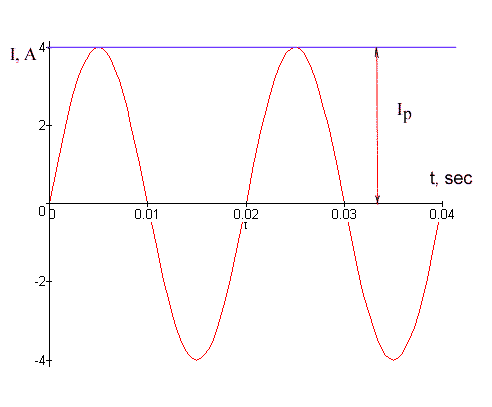Alternating Current (AC), Voltage, sinusoidal Waveform

# Alternating Current (AC), Voltage, sinusoidal Waveform,

Alternating current (AC) unlike Direct current (DC) flows first in one direction then in the opposite direction. Current amperage The is function of time. Direct current (DC) amperage is constant.

The most common AC waveform is a sine (or sinusoidal) waveform. See figure 1.Figure 1.

# Instantaneous Current and Voltagewhere  I = instantaneous current in amperes
Ip = the maximum, or peak, current in amperes
f - Frequency. The frequency is the number of cycles that is completed each second. Unit of measure: hertz (Hz)
t - time
Other notationq = the angular displacement in degrees or radians

The circuits illustrating all folowing electric circuits laws and circuit analysis techniques are included into Circuit Magic installation Visit main page to learn about Circuit Magic. (Symbolic electrical circuits simulation software to analyse circuits using Kirchhoff's Laws & other methods.)